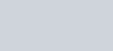# Data-Based and Statistical Reasoning

#### Measures of Central Tendency

• Mean: the average of the data. Is not outlier resistant
• Median: midpoint of data. If even number of data points, then median will be the average of two points. Outlier resistant.
• If mean and median far from each other, indicates presence of outliers or skewed distribution.
• Mode: number that appears the most often in a set of data.

#### Distributions

• Normal Distribution: can transform any normal distribution to a standard distribution with a mean of zero and a standard deviation one 1.
• Skewed Distribution: Contains a tail on one side of the data set and is thus not symmetric.
• Negative-Skewed: has a tail on the left, mean will be lower than the median
• Positively-skewed: has a tail on the right, mean will be larger than the median.
• Bimodal Distribution: Has two peaks, can sometimes be measured as two different distributions.

#### Measures of Distribution

• Range: difference between the largest and smallest values of a data set. Heavily affected by presence of data outliers. Standard deviation can be approximated as ¼ * range
• Interquartile Range: The third quartile minus the first quartile
• Quartiles: divide data into groups that comprise one-fourth of the entire data set.
• To calculate position of first quartile: sort data in ascending order and multiply n by 1/4
• If this is a whole number, the quartile is the mean of the value at this position and the next highest position
• If this is a decimal, round up to the next whole number and take that as the quartile position.
• For 3rd quartile, multiply n by 3/4. Do same process as first quartile.
• Outliers are those points that fall outside of 1.5*IQR
• Standard Deviation:• If data point falls more than three standard deviations from the mean, it is considered an outlier.
• On a normal distribution: 68-95-99 rule applies.
• Outliers: usually results from one of three causes:
• True statistical anomaly
• A measurement error
• Distribution is not approximated by a normal distribution.

#### Probability

• Mutually Exclusive Outcomes: cannot occur at the same time
• Exhaustive set of outcomes: no other possible outcomes.

#### Calculations

• For independent events, probability of two or more events occurring at the same time is the product of their probabilities alone
• The probability of at least one of two events occurring is equal to the sum of their initial probabilities minus the probability that will both occur.
•#### Statistical Testing

##### Hypothesis Testing
• Null Hypothesis: hypothesis of equivalence, says that two populations are equal.
• Alternative Hypothesis: non-direction (not equal) or direction (greater than or less than)
• Z-tests or t-tests are commonly used tests. Test Statistic is calculated form collected data, and compared to a table in order to determine the likelihood that the statistic was obtained by random choice. This likelihood is known as the p-value.
• If p-value > level of significance (usually 0.05) then the null hypothesis cannot be rejected.
• When null is rejected, results are statistically significant since there is a difference between the two groups.
• Level of significance is the level of risk that is accepted for incorrectly rejecting the null hypothesis. Also known as a type I error.
• Type I Error: Likelihood that we report a difference between the two population when one does not actually exist
• Type II Error: incorrectly fail to reject the null hypothesis. When no difference is reported when there actually is one. (b)
• Power: the probability of correctly rejecting the null hypothesis: 1-b
• Confidence: the probability of correctly failing to reject the null hypothesis when no difference exists.

##### Confidence Intervals
• Reverse of hypothesis testing, start off with a desired confidence (usually 95%) and use a table to find corresponding Z/t values. Scores are then multiplied by standard deviation and then added/subtracted from the mean

#### Charts, Graphs, and Tables

##### Types of Charts
• Pie/Circle Charts: represent relative amounts of entities. Loses impact as number of categories increases.
• Bar Charts and Histograms: Bar charts are used for categorical data, while histograms are for numerical data.
• Box Plots: used to show the range, median, quartiles and outliers for a set of data. Box-and-whisker is a labeled box plot.
• Box: bounded by Q1 and Q3, Q2 is the line in the middle (median).
• End of Whiskers: largest and smallest values in the data set that are not outliers.
• Maps: data is demonstrated geographically

##### Graphs and Axes
• Linear Graphs: can be linear, parabolic, exponential or logarithmic
• Axes of a linear graph will have units that occupy the same amount of space
•• Semilog and Log-Log Graphs: changes are made to one or both of the axis ratio’s.

#### Applying Data

• Correlation refers to a connection – direction relationship, inverse relationship, etc. – between data. This does not imply causation.

error: Content is protected !!